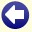## Solution to: To Know or not To Know

Answer: the three numbers are 36, 108, and 144. It is, however, not possible to determine which of the two numbers 36 and 108 Amy and Brad have.

Each of the three students sees two numbers and knows that his or her number is either the sum of these two numbers or the (positive) difference between these two numbers.

A student could know his or her number for sure if he or she sees two equal numbers (for example, if the three numbers would be 36, 36, and 72). In that case, his or her number must be the sum of the two other numbers. Since none of the students knows for sure what his or her own number is during the first round, none of them sees two equal numbers.

A student could also know his or her number for sure if the fact that one of the other students does not see two equal numbers means that one of the two possibilities for his or her own number is ruled out. In this case, the student sees a number x and a number 2x (for example, if the three numbers would be 36, 72, and 108). His or her own number can only be x or 3x (in the example: 36 or 108). However, if his or her number would be x, the student with the number 2x would have immediately figured out his or her own number (in the example, the three numbers would then have been 36, 72, and 36). So then, the only remaining possibility is 3x. Since none of the students knows for sure what his or her own number is after both other students have given their "I don't know" answer, none of them sees an x and a 2x.

A student could also know his or her number for sure if the fact that one of the other students does not see an x and a 2x means that one of the two possibilities for his or her own number is ruled out. In this case, the student sees a number x and a number 3x (for example, if the three numbers are 36, 108, and 144). His or her own number can only be 2x or 4x (in the example: 72 or 144). If his or her own number would be 2x, then the student with the number 3x would have figured that out after both other students have given their "I don't know" answer (in the example, the three numbers would then have been 36, 108, and 72).

Since Charles knows for sure what his number is directly after the two other students had their second turn, we conclude that Charles sees a number x and a number 3x and his own number is 4x. We know that 4x=144, so Charles sees the numbers 36 and 108.Back to the puzzle
This website uses cookies. By further use of this website, or by clicking on 'Continue', you give permission for the use of cookies. If you want more information, look at our cookie policy.